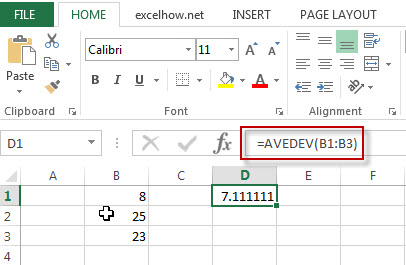## Excel AVEDEV Function

This post will guide you how to use Excel AVEDEV function with syntax and examples in Microsoft excel.

### Description

The Excel AVEDEV function returns the average of the absolute deviations of the numbers that you provided.

The AVEDEV function is a build-in function in Microsoft Excel and it is categorized as a Statistical Function.

The AVEDEV function is available in Excel 2016, Excel 2013, Excel 2010, Excel 2007, Excel 2003, Excel XP, Excel 2000, Excel 2011 for Mac.

### Syntax

The syntax of the AVEDEV function is as below:

= AVEDEV (number1, [number2],…)

Where the CHOOSE function arguments are:
Number1 -This is a required argument.  A numeric values

### Example

The below examples will show you how to use Excel AVEDEV Function to return a value from a value list based on a position value.

#1 To get the average of the absolute deviations of the numbers in cells B1:B3, just using the following excel formula: =AVEDEV(B1:B3)## Excel Statistical Functions

This section will learn  how to use Excel’s  Statistical Functions such as: Avedev, Average, AverageA, AverageIF, AverageIFS, etc.

AVEDEV – returns the average of the absolute deviations of the numbers that you provided.

AVERAGE – returns the average of the numbers that you provided

AVERAGEA – returns the average of its arguments, including numbers, text, and logical values.

AVERAGEIF – returns the average of all numbers in a range of cells that meet a given criteria.

AVERAGEIFS – returns the average of all numbers in a range of cells that meet multiple criteria.

BETA.DIST – calculate the cumulative beta distribution or beta probability density function.

BETADIST – returns the cumulative beta probability density function.

BETA.INV – returns the inverse of the beta cumulative probability density function.

BETAINV – returns the inverse of the beta cumulative probability density function.

BINOM.DIST – returns the individual term binomial distribution probability.

BINOM.INV – returns the inverse of the Cumulative Binomial Distribution that is greater than or equal to a criterion value.

BINOMDIST – returns the individual term binomial distribution probability.

CHIDIST – returns the right-tailed probability of the chi-squared distribution.

CHIINV – returns the inverse of the right-tailed probability of the chi-squared distribution.

CHITEST – returns the value from the chi-squared distribution for the statistic and the appropriate degrees of freedom.

COUNT – counts the number of cells that contain numbers, and counts numbers within the list of arguments.

COUNTA – counts the number of cells that are not empty in a range.

COUNTBLANK – use to count the number of empty cells in a range of cells.

COUNTIF – count the number of cells in a range that meet a given criteria.

COUNTIFS -returns the count of cells in a range that meet one or more criteria.

COVAR – returns covariance, the average of the products of deviations for each data point in two given sets of values.

FORECAST – used to calculate or predict a future value by using existing values.

FREQUENCY – calculates how often values occur within a range of values.

GROWTH – calculates the predicted exponential growth based on existing data.

INTERCEPT – calculates the point at which a line will intersect the y-axis by using existing x-values and y-values.

LARGE -returns the largest numeric value from the numbers that you provided. Or returns the largest value in the array.

LINEST – calculates the statistics for a line by using the “least squares” method to calculate a straight line that best fits your data, and then returns an array that describes the line.

MAX – returns the largest numeric value from the numbers that you provided.

MAXA – returns the largest numeric value from a range of values.

MEDIAN – returns the median of the given numbers.

MIN – returns the smallest numeric value from the numbers that you provided.

MINA -returns the smallest numeric value from the numbers that you provided, while counting text and the logical values.

MODE – returns the most frequently occurring number found in an array or range of numbers.

MODE.MULT – returns a vertical array of the most frequently occurring number found in an array or range of numbers.

MODE.SNGL -returns the most frequently occurring number found in an array or range of numbers.

PERCENTILE -returns the kth percentile from a supplied range of values.

PERCENTRANK – returns the rank of a value in a set of values as a percentage of the set.

PERMUT – returns the number of permutations for a given number of items.

QUARTILE – returns the quartile from a supplied range of values.

RANK – returns the rank of a given number in a supplied range of cells.

SLOPE – returns the slope of the linear regression line through data points in known_y’s and know_x’s.

SMALL -returns the nth smallest numeric value from the numbers that you provided.

STDEV – returns the standard deviation of a population based on a sample of numbers.

STDEVA – returns the standard deviation of a population based on a sample of numbers, text, and logical values.

STDEVP – returns the standard deviation of a population based on an entire population of numbers.

STDEVPA – returns the standard deviation of a population based on an entire population of numbers, text or logical values.

VAR – returns the variance of a population based on a sample of numbers.

VARA – returns the variance of a population based on a sample of numbers, text, or logical values.

VARP – returns the variance of a population based on an entire population of numbers.

VARPA – returns the variance of a population based on an entire population of numbers, text, or logical values.